V.5 No 2 21 On light aberrationThis unambiguously proves a simple fact that aberration is effected not due to relativistic speeds, it is caused by the feature of radiation and reception of waves of any kind in mutual motion of the source and receiver. Namely this important circumstance stipulates the negative result of Airy experiment, and we can easily show it, basing on the above classical derivation of the light aberration law. But first we would like to draw the Reader’s attention that from the view of relativistic approach to the results of Airy experiment, “if we consider the process from the view of accompanying, i.e. moving with the body’s system of coordinates, Airy’s result appears obvious. Actually, if we direct the tube to the place where seemingly is located the stationary star, the waves emitted by this last will incident on the objective normally. With the tube filled with water, the waves still will propagate perpendicularly to the separation surface. Thus, in the view of theory of relativity, Airy experiment considered from the Earth proves the trivial fact that with the perpendicular incidence, when the angle of incidence is zero, the angle of refraction is zero, too” [9, p. 169]. Doing not meanwhile deepen into the features of relativistic approach, we will only mark that Pauli’s estimation does not account a very simple circumstance that follows from the phenomenology of aberration. Actually, if the telescopic tube moves, then irrespectively of, whether the tube is directed to zenith or not, the beam direction will never coincide with the axis of telescope, since the beam needs some time to pass from the objective to the ocular. During this time the ocular will shift with the telescope. So, if we proceed from the fact that the negative result was caused just by the absence of refraction angle at the entrance of telescope filled with water, such approach will be surely incorrect. At the same time, should Airy’s result be caused namely by the refraction laws and by change of speed of beam propagation in water, this result would be positive. To show it, we have to follow Fig. 4.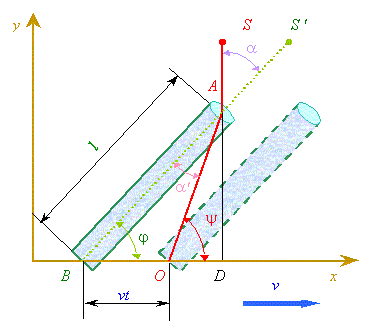Fig. 4. Airy experiment modelled with account of refraction angles and change of speed of light propagation in water
 We have to note the beam refraction at the entrance of telescope filled with water and that in this case the light will pass the section AO with the speed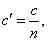(21)
 where n is the refraction coefficient of medium filling the telescope, since “as long ago as in 19th century there appeared the possibility to measure exactly the speed of light u in any substance (gaseous or liquid). From such measurements we can determine (21) and compare this value with the table value of refraction factor for this substance obtained from measurements based on the use of refraction law” [10, p. 44]. Noting these features, the parameters (4) of the main classical derivation will be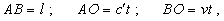(22)
 and the expression (5)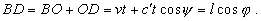(23)
 The time of beam passage from the objective to ocular will be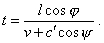(24)
 Substituting (24) to (7), we yield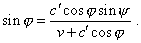(25)
 Noting that in the considered problem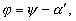(26)
 we will yield from (25)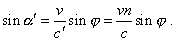(27)
 Now, to derive the interrelation of real aberration angle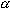with the observation angle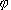, we have to take into account the beam refraction at the entrance of telescope. In accordance with Snell - Descartes law  (the sine law),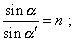(28)
 hence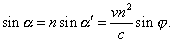(29)
 Thus, if we calculate on the basis of light refraction law and of change of speed of light in water, then, just as Airy predicted, he would have obtained a considerable effect - in proportion to the water refraction coefficient squared, which is about 2, 7. And Airy would surely register the difference, if not the feature of geometrical optic of telescopic systems, which he did not take into account. It is known that “in modern visual refractors, Kepler ocular is used. The magnification of such instrument is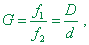(30)
 where f1 and f2 are, correspondingly, the focal distances of the objective and ocular, and D and d are, accordingly, the diameters of input and output pupils of the refractor. With the given diameter of objective D, the maximal magnitude G is limited by the output pupil, which has to be no less than 0,7 mm; larger magnification will lower the brightness and contrast of images” [12, p. 441]. With it, “a beam of parallel rays coming from an infinitely distanced source converges at so-called back focus of the lens” [7, p. 52]. If the refraction coefficients of medium before and after lenses of objective and ocular of the tube were different, after the laws of geometrical optics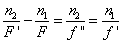(31)
 (where f' and f'' are the front and back focal distances, F is the distance to the object, F' is the distance to the image,  n1 and n2 are the refraction coefficients of media before and after the lens), we will yield for the objective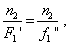(32)
 where  f ''1 is the back focal distance of the objective, and F'2 is the distance from object to the image [7, p. 53] For the ocular, we yield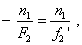(33)
 where f '2 is the front focal distance of the ocular, and F2 is the distance from the object imaginary for the ocular - to the ocular [7, p. 53]. Thus, irrespectively of, whether the telescope was filled with water,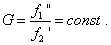(34)
 Notice that in order to make his results comparable, Airy had to establish the equal magnification G; the aberration angle change was simply masked when the telescope was filled with water, because of features of geometrical optics of the very telescopic system. This feature did not affect the aberration angle itself, as when the beam was inclined to the telescope axis, the inclination angles of incidenting beam w1 and direction of view w2   give a very simple relation (see Fig. 5) [4, p. 89], [7, p. 65]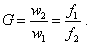(35)
 Astronomers take this feature into account when determining the Earth hodograph.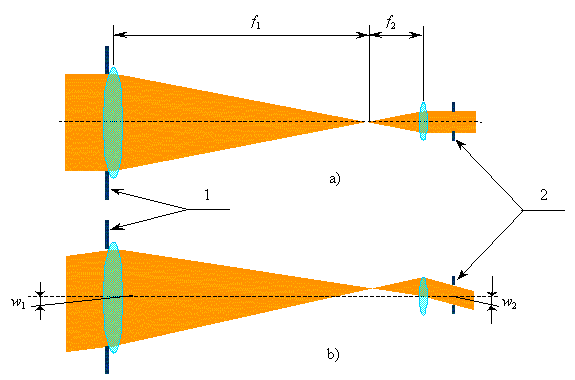Fig. 5. “The demonstration experiment to illustrate the telescopic beam path in Kepler tube for a remote point of the object located on the axis of lenses (a) and out of this axis (b)” [4, Fig. 101, p. 88]: 1 is the input pupil and 2 is the output pupil
 This feature of telescopic system is revealed also in Fig. 5b, where with the beam’s inclined fall on the objective, it propagates within the telescope not along the direct line. At small speeds of the body, this has a little effect on the result of measurement, but at large speeds (which is especially important from the view of general theory of phenomenon) this deviation will change the final result of calculation. Basically, it is not hard to account this nuance, determining more precisely in (4) and (6) the distance, which the beam passes in the tube. However, this exceeds the limits of present problem - to compare the classical and relativistic approaches in the most general, phenomenological form; this is rather a metrological problem related to the refinement of methods to measure the Earth hodograph. But if speaking of Airy experiment, as we have it cleared up, the result was negative not because in classical view the water-filling of telescope tube has no effect on the result of measurement, but because of masking effect caused by the features of optical system of telescope. And it is easy to check it, if in order to test the aberration effect we choose the scheme, whose principles of construction we will discuss after the analysis of relativistic view on aberration effect.

Contents: / 18 / 19 / 20 / 21 / 22 / 23 / 24 / 25 / 26 / 27 / 28 /